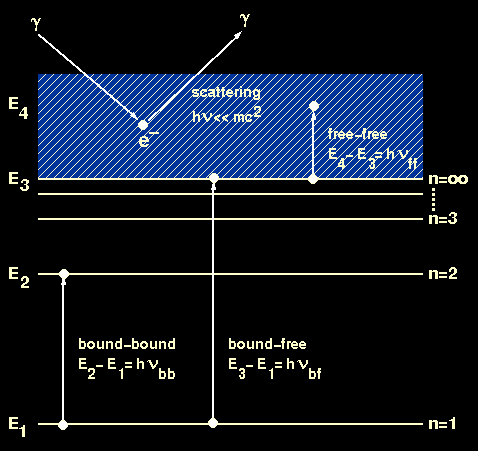We first introduced the concept of opacity when deriving the equation of radiative transport. Opacity is the resistance of material to the flow of heat, which in most stellar interiors is determined by all the processes which scatter and absorb photons. We will now look at each of these processes in turn, of which there are four:
• bound-bound absorption
• bound-free absorption
• free-free absorption
• scattering
The first three are known as true absorption processes because they involve the disappearance of a photon, whereas the fourth process only alters the direction of a photon. All four processes are described below and are shown pictorially in figure 12.

 Figure 12: Schematic energy level diagram showing the four microscopic processes which contribute to opacity in stellar interiors.bound-bound absorption

Bound-bound absorptions occur when an electron is moved from one orbit in an atom or ion into another orbit of higher energy due to the absorption of a photon. If the energy of the two orbits is E1 and E2, a photon of frequencybb will produce a transition if

E2 - E1 = hbb.

Bound-bound processes are responsible for the spectral lines visible in stellar spectra, which are formed in the atmospheres of stars. In stellar interiors, however, bound-bound processes are not of great importance as most of the atoms are highly ionised and only a small fraction contain electrons in bound orbits. In addition, most of the photons in stellar interiors are so energetic that they are more likely to cause bound-free absorptions, as described below.

bound-free absorption

Bound-free absorptions involve the ejection of an electron from a bound orbit around an atom or ion into a free hyperbolic orbit due to the absorption of a photon. A photon of frequencybf will convert a bound electron of energy E1 into a free electron of energy E3 if

E3 - E1 = hbf.

Provided the photon has sufficient energy to remove the electron from the atom or ion, any value of energy can lead to a bound-free process. Bound-free processes hence lead to continuous absorption in stellar atmospheres. In stellar interiors, however, the importance of bound-free processes is reduced due to the rarity of bound electrons.

free-free absorption

Free-free absorption occurs when a free electron of energy E3 absorbs a photon of frequencyff and moves to a state with energy E4, where

E4 - E3 = hff.

There is no restriction on the energy of a photon which can induce a free-free transition and hence free-free absorption is a continuous absorption process which operates in both stellar atmospheres and stellar interiors. Note that, in both free-free and bound-free absorption, low energy photons are more likely to be absorbed than high energy photons.

scattering

In addition to the above absorption processes, it is also possible for a photon to be scattered by an electron or an atom. One can think of scattering as a collision between two particles which bounce of one another. If the energy of the photon satisfies

h<< mc2,

where m is the mass of the particle doing the scattering, the particle is scarcely moved by the collision. In this case the photon can be imagined to be bounced off a stationary particle. Although this process does not lead to the true absorption of radiation, it does slow the rate at which energy escapes from a star because it continually changes the direction of the photons.